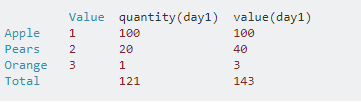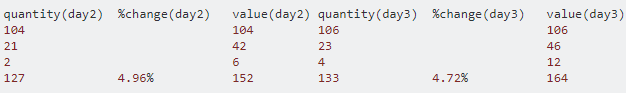2 views

I have a problem with a game I am making. I think I know the solution(or what solution to apply) but not sure how all the ‘pieces’ fit together.

How the game works:

users will be given items with a value(values change every day and the program is aware of the change in price). For example

apple=1 pears=2 oranges=3

They will then get a chance to choose any combo of them they like (i.e. 100 apples, 20 pears, and 1 orange). The only output the computer gets is the total value(in this example, its currently \$143). The computer will try to guess what they have. Which obviously it won’t be able to get correctly the first turn.The next turn the user can modify their numbers but no more than 5% of the total quantity (or some other percent we may choose. I’ll use 5% for example.). The prices of fruit can change(at random) so the total value may change based on that also(for simplicity I am not changing fruit prices in this example). Using the above example, on day 2 of the game, the user returns a value of \$152 and \$164 on day 3. Here's an example.I am trying to see if I can figure out what the quantities are over time(assuming the user will have the patience to keep entering numbers). I know right now my only restriction is the total value cannot be more than 5% so I cannot be within 5% accuracy right now so the user will be entering it forever.

What I have done so far:

I have taken all the values of the fruit and total value of the fruit basket that’s given to me and created a large table of all the possibilities. Once I have a list of all the possibilities I used graph theory and created nodes for each possible solution. I then create edges(links) between nodes from each day(for example day1 to day2) if it’s within a 5% change. I then delete all nodes that do not have edges(links to other nodes), and as the user keeps playing I also delete entire paths when the path becomes a dead end. This is great because it narrows the choices down, but now I’m stuck because I want to narrow these choices even more. I’ve been told this is a hidden markov problem but a trickier version because the states are changing(as you can see above new nodes are being added every turn and old/non-probable ones are being removed).

What I think needs to be done(this could be wrong):

Now that I narrowed the results down, I am basically trying to allow the program to try to predict the correct based the narrowed result base. I thought this was not possible but several people are suggesting this can be solved with a hidden markov model. I think I can run several iterations over the data(using a Baum-Welch model) until the probabilities stabilize(and should get better with more turns from the user). The way hidden markov models are able to check spelling or handwriting and improve as they make errors(errors, in this case, is to pick a basket that is deleted upon the next turn as being improbable).

Two questions:

1. How do I figure out the transition and emission matrix if all states are at first equal? For example, as all states are equally likely something must be used to dedicate the probability of states changing. I was thinking of using the graph I made to weight the nodes with the highest number of edges as part of the calculation of transition/emission states? Does that make sense or is there a better approach?

2. How can I keep track of all the changes in states? As new baskets are added and old ones are removed, there becomes an issue of tracking the baskets. I though the Hierarchical Dirichlet Process hidden markov model(hdp-hmm) would be what I needed but not exactly sure how to apply it.

As always, thanks for your time and any advice/suggestions would be greatly appreciated.

by (108k points)

As you have mentioned above that you are using the Hidden Markov Model approach for narrowing your results to get a specified path. In simpler Markov models, the state is directly visible to the observer, and therefore the state transition probabilities are the only parameters, while in the hidden Markov model(HMM), the state is not directly visible, but the output depending on the state, is visible. Each state has a probability distribution over the possible output tokens(data). Therefore, the sequence of tokens(data) generated by an HMM gives some information about the sequence of states which is also known as pattern theory.

Now comes finding the most likely path:

For finding the most likely path we will follow the Viterbi algorithm:

The idea is to use dynamic programming to compute for every state k and every position I in the sequence the value.

The algorithm goes as follows:

Initialization:

v0(0)=1;

v k(0)=0;

for k >0;

• Recursion:

for i= 1 to t for all states k

vk(i)= e(k,σi )maxj vj(i-1)a(j,k)

trace-back for path determination:

trace(k,i) = record the source of the arrow that gives maxj vj(i-1)a(j,k)

P*(t) =trace(stop,t);

P(t) is predecessor of state t

• Trace-back:

for i = t to 1 do P*(i-1) = trace(P*(i),i)

Now we can compute quickly the most likely path (and the probability of this path).

Coming back to your question, if you want to figure out the transition and emission matrix if all states are equal at first, you have to define all possible events related to your problem. Then divide the data into columns containing all the possibilities.

Transition probability for the same states will be the frequency of transition from the event of one state to the second state divided by all transitions from the event of one state to all other states.

Want to master Machine Learning? Sign up for this Machine Learning Certification course!